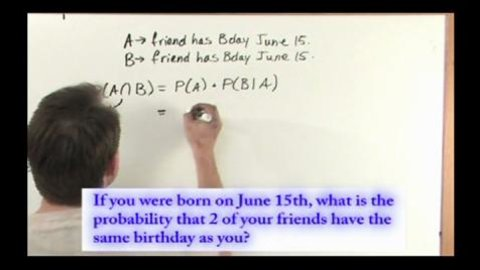# Conditional Probability Part of the Series: Probability and Statistics Tutor Series

##### TMW Media## Related videos

Probability and Statistics Tutor Series
The Probability and Statistics Tutor is a complete 14 lesson series covering all of the core topics in detail. What sets this series apart from other teaching tools is that the concepts are taught entirely through step-by-step example problems of increasing difficulty. It works by introducing each new concept in…
Fundamentals of Probability
This program covers the important topic the fundamentals of probability in Probability and Statistics. We begin by discussing what the concept of probability really means and why it is important. Next, we solve several problems that involve the essential ideas of probability to give students practice with the material.
This program covers the important topic of the addition rules of probability in Probability and Statistics. We begin by discussing what the addition rules of probability really mean and why they are important. Next, we solve several problems that involve the essential ideas of addition in probability to give students…
Random Variables and Introduction to Probability Distributions
This program covers the important topic of Random Variables and Probability Distributions in Probability and Statistics. We begin by discussing what a random variable is and how it applies to probability distributions.
Binomial Probability Distribution
This program covers the important topic of the Binomial Probability Distribution in Probability and Statistics. We begin by discussing what the Binomial Distribution is and why it is important. Next, we solve several problems that involve the Binomial Distribution to give students practice with the material.
Poisson Probability Distribution
This program covers the important topic of The Poisson Probability Distribution in Probability and Statistics. We begin by discussing what the Poisson Distribution is and why it is important. Next, we solve several problems that involve this important distribution to give students practice with the material.
Normal Probability Density
This program covers the important topic of the Normal Probability Density in Probability and Statistics. We begin by discussing what the Normal Density is and why it is important. Next, we solve several problems that involve the Normal Probability Density to give students practice with the material.
Probability
Expressing a probability between certainty and impossible as the likelihood of an uncertain event occurring --conducting and recording probability experiments using small and large number trials with replacement --calculating the theoretical probability of a particular event occurring --expressing probabilities as odds
Inverse Trigonometric Functions
This program covers the important topic of Inverse Trigonometric Functions in Calculus. We begin by discussing what an inverse trigonometric function is and why it is a central topic in Calculus. Next, we solve several practical calculus problems that give students practice with inverse trigonometric functions. The entire lesson is…
Derivatives of Inverse Trigonometric Functions
This program covers the important topic of Derivatives of Inverse Trigonometric Functions in Calculus. We begin by discussing what an inverse trigonometric function is, how to take its derivative, and why it is a central topic in Calculus. Next, we solve several practical calculus problems that give students practice with…
Hyperbolic Functions
This program covers the important topic of Hyperbolic Functions in Calculus. We begin by discussing what a Hyperbolic function is and why it is a central topic in Calculus. Next, we solve several practical calculus problems that give students practice with Hyperbolic functions. The entire lesson is taught by working…
Inverse Hyperbolic Functions
This program covers the important topic of Inverse Hyperbolic Functions in Calculus. We begin by discussing what an Inverse Hyperbolic function is and why it is a central topic in Calculus. Next, we solve several practical calculus problems that give students practice with Inverse Hyperbolic functions. The entire lesson is…